# Understanding alkali metal cation affinities of multi-layer guanine quadruplex DNA†

C. Nieuwlanda, F. Zaccariaa and C. Fonseca Guerra*ab
aDepartment of Theoretical Chemistry and Amsterdam Center for Multiscale Modelling, AIMMS, Vrije Universiteit Amsterdam, De Boelelaan 1085 NL-1081HV Amsterdam, The Netherlands. E-mail: c.fonsecaguerra@vu.nl
bLeiden Institute of Chemistry, Leiden University, Einsteinweg 55 NL-2333 CC Leiden, The Netherlands

Received 26th June 2020 , Accepted 9th September 2020

First published on 10th September 2020

To gain better understanding of the stabilizing interactions between metal ions and DNA quadruplexes, dispersion-corrected density functional theory (DFT-D) based calculations were performed on double-, triple- and four-layer guanine tetrads interacting with alkali metal cations. All computations were performed in aqueous solution that mimics artificial supramolecular conditions where guanine bases assemble into stacked quartets as well as biological environments in which telomeric quadruplexes are formed. To facilitate the computations on these significant larger systems, optimization of the DFT description was performed first by evaluating the performance of partial reduced basis sets. Analysis of the stabilizing interactions between alkali cations and the DNA bases in double and triple-layer guanine quadruplex DNA reproduced the experimental affinity trend of the order Li+< Rb+ < Na+ < K+. The desolvation and the size of alkali metal cations are thought to be responsible for the order of affinity. Nevertheless, for the alkali metal cation species individually, the magnitude of the bond energy stays equal for binding as first, second or third cation in double, triple and four-layer guanine quadruplexes, respectively. This is the result of an interplay between a decreasingly stabilizing interaction energy and increasingly stabilizing solvation effects, along the consecutive binding events. This diminished interaction energy is the result of destabilizing electrostatic repulsion between the hosted alkali metal cations. This work emphasizes the stabilizing effect of aqueous solvent on large highly charged biomolecules.

## 1. Introduction

DNA sequences that are purine rich and contain runs of guanine (G) bases can form non-duplex four-stranded structures that are called guanine quadruplexes (GQs) (Fig. 1).1–5 GQs consist of stacked guanine quartets with alkali cations between the layers and occur in crucial regulatory regions of the human genome, such as telomeres and promotors. The guanine quartet (G4) layers consists of four guanine bases assembled through Hoogsteen-type hydrogen bonding (Fig. 1a). The stabilization of GQ structures in telomeric regions has been shown to interfere with the activity of the enzyme telomerase, offering a tool to control the mechanism of elongation of telomeric ends that is related to cell senescence and therefore proliferation. On the other hand, the formation of GQ structures in telomeric and promotor regions of numerous genes can down-regulate transcription and suppress oncogene expression. Therefore, GQs have been considered as an attractive target for anticancer drug design.6–9 This potential therapeutic application has led to a new field devoted to the design of (metallo)organic compounds that selectively interact, promote and stabilize the GQ folding.10–12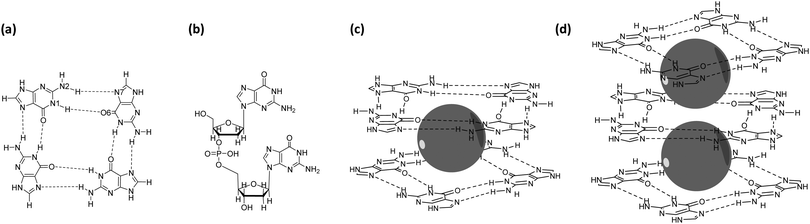Fig. 1 Structural representation of (a) a guanine quartet (G4) with inner (N1(H)⋯O6) and outer (N2(H)⋯N7) hydrogen bonds specified, (b) a guanine dimer (GG) with sugar-phosphate backbone neutralized with H+ as counterion, (c) a guanine quadruplex double-layer scaffold with an alkali metal ion presented as a grey sphere (G4–M+–G4) and (d) a guanine quadruplex triple-layer scaffold with two alkali metal ions (G4–M+–G4–M+–G4).

However, in order to rationally design these so-called GQ-ligands, it is crucial to fully understand the physical principles behind the formation of GQs and the role of the alkali cations in the stabilization of these structures.

Previous work by Fonseca Guerra et al.13,14 has shown that the hydrogen bonding in G4 relies on cooperativity. These synergistic effects originate from charge separation occurring upon hydrogen bonding, due to donor–acceptor interactions of the σ-electron system.15 It contradicts previous assumptions that the enhancement of the hydrogen bonds was solely caused by resonance of the π-electrons. In addition, Fonseca Guerra and co-workers demonstrated that although the presence of alkali metal cations between the G4 layers weakens the hydrogen bonds, the synergy remains in telomeric structures.16–19

The alkali metal cation that is located between two quartets was long believed to generate cation–dipole interactions with the eight interacting guanines and thereupon reducing the repulsion of the central oxygen atoms.20–22 This would enhance the hydrogen bonding strength and stabilize the GQ formation. This idea was rationalized by the presence of significant negative charge located at the central cavity of the G4.21 In 2016, work by Zaccaria et al.23 revealed that the central cation is not needed to reduce repulsion between the oxygen atoms, but rather provides additional stability of the quadruplex by electrostatic and donor–acceptor interactions between the oxygens and the cation. Later work showed that inclusion of the metal cation into the quadruplex significantly lowers the Gibbs free energy of formation of the cationic species compared to the formation of the empty scaffold.24 This led to the insight that the actual need for the cation is to overcome the entropic penalty.

Guanine quadruplexes can host different alkali metal cations, and many experimental studies have revealed an overall affinity sequence of K+ > Na+, Rb+ ≫ Li+, Cs+ in water.25–27 Research has been devoted to finding an explanation of this affinity trend.28–30 Williamson et al.28 suggested in 1989 that the overserved ion specificity was related to the snug fit of the alkali cations into the cavity of the quadruplex, thus the size of the cations. Later, Hud and co-workers30 claimed that the Gibbs free energy of solvation of the alkali metal cations was the determining factor, since cations have to be desolvated completely before they can access the inner channel of GQs. This idea was supported by the theoretical work of Gu and Leszczynski.29

Zaccaria et al.23 showed that both the ionic radius, as well as the Gibbs free energy of solvation, are of equal importance in determining the order of cation affinity in GQ structures. These results were obtained from extensive computational analysis of the electronic properties of double-layer GQ structures interacting with the different alkali metal cations. These computations were based on dispersion-corrected density functional theory (DFT-D).31–36 The study of Zaccaria and co-workers involved double-layer quadruplexes (Fig. 1c). However, natural occurring GQ structures can be encountered as multiple stacks of G4.4,7,37 Therefore, we have investigated if our previous results and conclusions can be extended to larger systems which include interactions between adjacent cations.

In this work we investigate the electronic interactions of double, triple and four-layer GQ structures (with and without sugar-phosphate backbone) with the different alkali metal cations in aqueous solution by computational analysis using DFT-D based calculations. To facilitate the computations on these significant larger systems, optimization of the DFT description was performed at first by evaluating the performance of partial reduced basis sets. In case of the triple-layer systems, the computational geometries involve quadruplex structures occupied by one potassium cation, to which a second alkali metal cation is introduced. The four-layer GQ comprises a structure hosting three potassium cations. The calculations simulate the situation of quadruplexes under supramolecular and biological conditions in aqueous solution.

## 2. Computational methods

### 2.1 General procedure

All the calculations were performed using the Amsterdam Density Functional (ADF) program (ADF2017.103).38–40 Geometry optimizations and stationary point energy calculations were based on dispersion-corrected relativistic density functional theory at the ZORA-BLYP-D3(BJ) level of theory.31–36,41 A TZP basis set was used for the description of the alkali metal cations and the guanine bases, whereas DZ was used as basis set for the sugar phosphate backbone. Our benchmark study revealed that the usage of these smaller basis sets is justified (see Section 3.1 and ESI Fig. S1 and S2). The use of the BLYP exchange functional in combination with D3 London dispersion correction with Becke–Johnson damping is supported by previous computational work on guanine quadruplexes that demonstrated that this description results in reliable geometries and trends in line with experiment.23,24,35,42 In addition, Sedlak et al. showed that BLYP-D3(BJ) outperforms semi-empirical functionals, such as M06-2X, in terms of accuracy:cost ratio for the description of non-covalent interactions.43 Because of this combination of high accuracy and low computational costs, BLYP-D3(BJ) was used in this work.

No geometrical constraints were imposed on the optimized geometries. This also applied to the guanine quadruplexes without backbone (129 atoms (2-layer), 194 atoms (3-layer) and 259 atoms (4-layer)) and the guanine quadruplex with backbone (265 atoms (2-layer) and 402 atoms (3-layer)). The guanine quadruplexes in this work are parallel stranded righthanded GQ structures with anti-glycosidic torsion angles at all guanines. Biological GQ structures are also constituted by loop regions with dangling nucleobases. However, dangling nucleobases do not have significant influence on binding properties of the shielded interior of the quadruplex and are therefore omitted in the model systems.

Optimized geometries of the double-layer GQ structures at the ZORA-BLYP-D3 (BJ)/TZ2P level of theory of Zaccaria et al. were used as starting point for the calculations of the triple-layer systems.23,24 Solvent effects in water have been taken into account with the conductor-like screening model (COSMO), as implemented in the ADF program.36,44–46 Radii of the cations have been determined by reproduction of the solvation energy of the cation according to the procedure presented in ref. 36 (see ESI, Table S1). In ADF releases after ADF2016 an improved method to construct the solvation surface in COSMO (Delley cavity construction instead of the Solvent-Excluding-Surface (SES) method) was implemented that led to more reliable geometry optimizations when using the COSMO solvation model.40 To compare the results of the double-layer quadruplexes with the triple-layer systems, the results of Zaccaria et al. were reproduced using the improved solvation model. Energies regarding the double-layer GQs where calculated as formulated by Zaccaria and co-workers.23

The energy of formation, ΔEform, of the triple-layer guanine quadruplex structures is defined by eqn (1a) and (1b), for the GQ without and with sugar-phosphate backbone, respectively (see also Fig. 2).

 ΔEform = E(G4–K+–G4–M+–G4) − 12·E(G) − E(K+) − E(M+) (1a)
 ΔEform = E(GQ–K+–M+) − 4·E(GGG) − E(K+) − E(M+) (1b)
E(G) is the energy of the separately optimized geometry of the guanine monomer. E(GGG) refers to the energy of the optimized guanosine trimer, wherein the sugar-phosphate backbone is neutralized by H+ as counterion (Fig. 1b). Zacceria et al. found that neutralization with H+ gives the same affinity trend as with neutralizion with Na+.23E(M+) denotes the energy of the central alkali metal cation (i.e. Li+, Na+, K+ or Rb+). E(G4–K+–G4M+–G4) and E(GQ–K+M+) are the energies of the triple-layer GQs, without and with backbone, in the optimal geometry.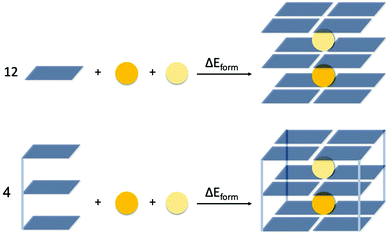Fig. 2 Definition of the formation energy (ΔEform) of the triple-layer guanine quadruplex structures without (G4, top scheme) and with sugar-phosphate backbone (GQ, bottom scheme).

### 2.2 Bond energy analysis

The bond energy between the second alkali metal cation and the triple-layer GQ structures, with one cavity occupied by K+ and one empty cavity, is formulated by eqn (2a) and (2b) for the guanine quadruplexes without (G4) and with backbone (GQ), respectively (see also Fig. 3).
 ΔEbond = E(G4– K+–G4– M+–G4)aq − E(G4–K+–G4–[ ]–G4)aq − E(M+)aq (2a)
 ΔEbond = E(GQ–K+–M+)aq − E(GQ–K+–[ ])aq − E(M+)aq (2b)
In these equations E(G4–K+–G4M+–G4)aq and E(GQ–K+M+)aq are the energies of the in water optimized triple-layer GQs, without and with backbone, occupied by one potassium cation and another alkali metal cation (M+ = Li+, Na+, K+ or Rb+). E(G4–K+–G4–[ ]–G4)aq and E(GQ–K+–[ ])aq denote the energies of the optimized triple-layer GQs in water, without and with backbone, occupied by one potassium cation.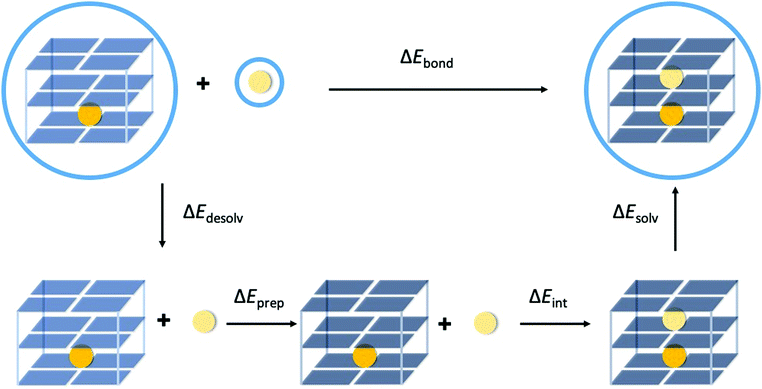Fig. 3 Partitioning of the bond energy between the second alkali metal cation (light yellow sphere) in the empty cavity of the by K+ (orange sphere) occupied triple-layer guanine quadruplex structure. The blue circles surrounding the structures indicate that the calculation is performed with COSMO to simulate water.

In order to understand the different components that determine the trend in bond energy (i.e. cation affinity) the bond energy was partitioned as formulated by eqn (3) (see also Fig. 3).

 ΔEbond = ΔEdesolv + ΔEprep + ΔEint + ΔEsolv (3)
The desolvation (ΔEdesolv) and solvation energy (ΔEsolv) can be computed by taking the energy difference between the solvated structure and the gas phase single-point calculation of the solvated optimized geometry (see eqn (4) and (5)). The subscript ‘aq’ indicates calculations performed with COSMO, whereas the subscript ‘gas’ refers to gas phase calculations.
 ΔEdesolv = E(G4–K+–G4–[ ]–G4)gas − E(G4–K+–G4–[ ]–G4)aq + E(M+)gas − E(M+)aq (4a)
 ΔEdesolv = E(GQ–K+–[ ])gas − E(GQ–K+–[ ])aq + E(M+)gas − E(M+)aq (4b)
 ΔEsolv = E(G4–K+–G4–M+–G4)aq − E(G4–K+–G4–M+–G4])gas (5a)
 ΔEsolv = E(GQ–K+–M+)aq − E(GQ–K+–M+)gas (5b)
The preparation energy (ΔEprep) is the energy required to deform the solvated state geometry of the triple-layer GQ structure, with one cavity occupied by K+ and one empty cavity, (G4–K+–G4–[ ]–G4 or GQ–K+–[ ]) to the geometry it acquires when it interacts with the second alkali metal cation, but calculated in the gas phase (see Fig. 3). The values of the preparation have been checked to be almost phase independent (see ESI, Table S2). The procedure to determine ΔEprep in the gas phase has been chosen to not have contributions by the implicit solvent in this energy term.

Finally, the interaction energy (ΔEint) can be computed in the gas phase for the geometries of the solvated state (eqn (6a) and (6b)).

 ΔEint = E(G4–K+–G4–M+–G4)gas − E(G4–K+–G4–[ ]–G4)gas − E(M+)gas (6a)
 ΔEint = E(GQ–K+–M+)gas − E(GQ–K+–[ ])gas − E(M+)gas (6b)
This approximation can be made since the interaction of the alkali metal cation occurs on the interior of the quadruplex cavity, which is shielded from the solvent by the guanine bases and sugar-phosphate backbone. In addition, it assumed that the negatively charged central cavity prevents entering of water molecules.1

The interaction energy is further decomposed based on the Kohn–Sham molecular orbital theory using a quantitative energy decomposition analysis (EDA), which divides the total interaction energy (ΔEint) into electrostatic interaction (ΔVelstat), Pauli repulsion (ΔEPauli), orbital interaction (ΔEoi) and dispersion correction (ΔEdisp) components (eqn (7)).47

 ΔEint = ΔVelstat + ΔEPauli + ΔEoi + ΔEdisp (7)
ΔVelstat corresponds to the classical electrostatic interactions between the unperturbed charge distributions of the prepared (i.e. deformed) interacting molecular fragments and is usually attractive. ΔEPauli comprises the destabilizing interactions between the occupied orbitals and accounts for any steric repulsion. The term ΔEoi includes charge transfer (i.e. donor–acceptor interactions between occupied orbitals on one of the interacting fragments and unoccupied orbitals on the other, including the HOMO–LUMO interactions) and polarization (empty-occupied orbital mixing on one fragment due to the presence of the other fragment). The term ΔEdisp includes dispersion corrections.

### 2.3 Voronoi deformation density analysis

The Voronoi deformation density (VDD) method allows analysis of the electronic redistributions within polyatomic fragments when a chemical bond is formed between these molecular fragments.48,49 The VDD atomic charges (ΔQM+) have been calculated for the second alkali metal cations in the central cavity of the triple-layer quadruplex (GQ–K+M+). ΔQM+ is computed as the (numerical) integral of the deformation density (Δρ(r)) in the volume of the Voronoi cell of cation M+ associated with the formation of the triple-layer quadruplex from the quadruplex hosting one K+ and the second metal cation (see eqn (8)). The Voronoi cell of M+ is the space defined by the bond midplanes on and perpendicular to all bond axes between nucleus M+ and its neighbouring nuclei.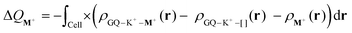(8)
ΔQM+ is an indication for the number of electrons that flows into (ΔQM+ < 0) or out of (ΔQM+ > 0) the Voronoi cell of M+ as result of the interaction of M+ with GQ–K+–[ ]. Cell is the Voronoi Cell of M+ in GQ–K+M+.

## 3. Results and discussion

### 3.1 Basis set dependency

Since DNA-based systems, especially including metals, encounter many non-covalent interactions, the use of triple-zeta (or larger) basis sets is often recommended when studying these systems.50 However, in these large (supramolecular) biomolecules, large basis sets can make the calculations too expensive. Therefore, smaller basis sets, which would combine high computational speed and accuracy, are preferred. The performance of the basis sets SZ, DZ, DZP, TZP and TZ2P and combinations of these for different regions of the quadruplexes were screened by stationary point calculations on the optimized double-layer GQ geometries at the ZORA-BLYP-D3(BJ)/TZ2P level of theory of Zaccaria et al. (see ESI Fig. S1 and S2).23 By comparing the obtained energies with the energy of the TZ2P calculation, the most promising (i.e. lowest computational cost, with sufficient accuracy (≤1 kcal mol−1 deviation)) basis set combination was selected. The results show that usage of different basis sets within the ring system of the guanine base, leads to significant errors in calculated energies (ESI, Fig. S1). This result is in accordance to the results found by Fonseca Guerra et al. in 1999.51 In this work it was found that hydrogen bonding is not a local effect, but affects the distributions of the σ and π electrons throughout the whole DNA base. The results of this work show that the basis set combination using TZP for the guanine bases and K+, in combination with a DZ basis set for the sugar–phosphate backbone gives a ΔEform within the 1 kcal mol−1 deviation range and seems to be sufficient to describe quadruplexes (Table 1 and ESI, Fig. S2). To validate this, the performance of the TZP/DZ basis set combination was examined in a geometry optimization of GQ-K+. The results of these calculations are depicted in Table 1. Both the energy and the geometrical parameters are in good accordance with the by TZ2P obtained results. Nevertheless, the average calculation time per cycle is almost three times shorter. Therefore, using the TZP/DZ basis set combination is likely to significantly reduce the calculation time on DNA based systems, compared to the conventional TZ2P basis set, while maintaining the required computational accuracy. The TZP/DZ basis set combination was used in the calculations of the quadruplexes throughout this work.
Table 1 Performance of DFT-D ZORA-BLYP-D3(BJ)/TZP/DZ in the geometry optimization of GQ–K+ in the gas phase
TZ2P TZP/DZ
a The energies (in kcal mol−1) were computed with the DFT-D method at the ZORA-BLYP-D3(BJ) level of theory in the gas phase. b Average distance (in Å) between the oxygen atoms and the midpoint of the eight oxygen atoms. c Difference in the average z-coordinate (in Å) of the upper and lower oxygen atoms. d Average outer hydrogen bond distance N2(H)⋯N7 (in Å). e Average inner hydrogen bond dissonance N1(H)⋯O6 (in Å). f Average OPO angle (in degrees) of bridging two sugar molecules of the backbone.
Calculated ΔEforma −277.3 −277.0
Relative average calculation time/geometry optimization cycle 1 0.3
d[O–K+]b 2.81 2.81
R 3.12 3.11
N2(H)⋯N7d 2.90 2.90
N1(H)⋯O6e 2.81 2.80
∠OPOf 107.0 106.1

### 3.2 Structure and energy of formation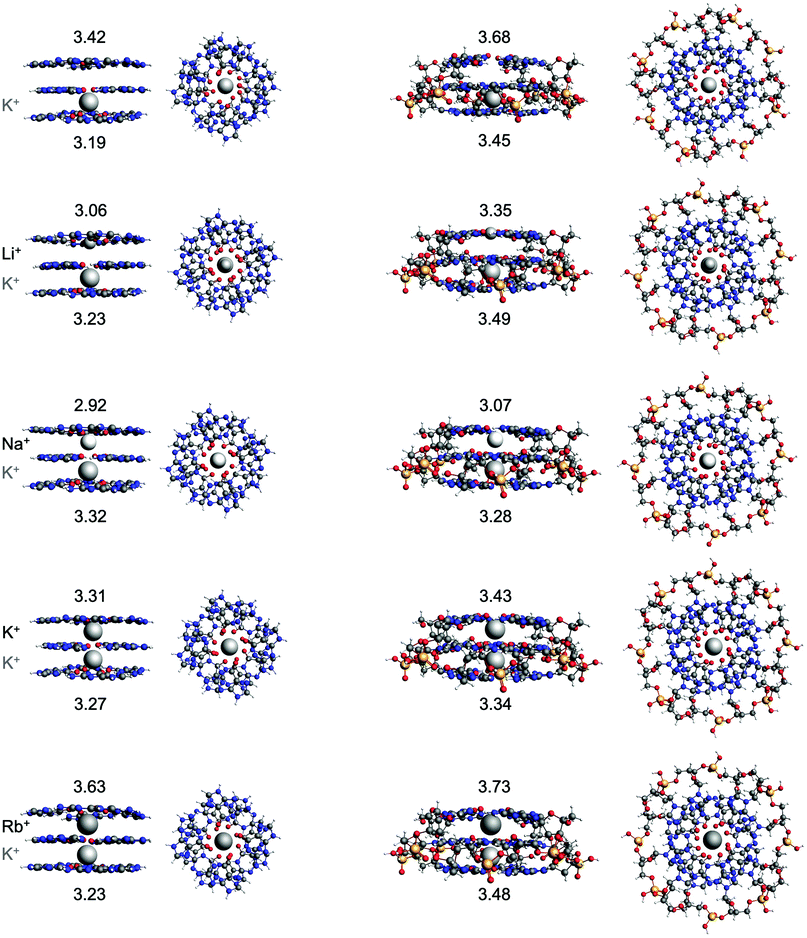Fig. 4 Structures of G4–K+–G4–[ ]–G4, G4–K+–G4–M+–G4, GQ–K+–[ ] and GQ–K+–M+ where M+ is an alkali metal cation. The G4 structures were optimized at the ZORA-BLYP-D3(BJ)/TZP level of theory and the GQ structures at the ZORA-BLYP-D3(BJ)/TZP/DZ level of theory with COSMO to simulate water. The difference in the average z-coordinate of the upper and lower oxygen atoms (R, in Å), as measure for the interplanar distance of adjacent guanine quartets, is displayed.
Table 2 Energies of formationa (in kcal mol−1) of the double- and triple-layer guanine quadruplexes and geometrical parameters (in Å) of the layer hosting M+. The geometrical parameters of the layer hosting K+ are shown in parentheses in case of the triple-layer systems
System M + d[O–M+]b R N2(H)⋯N7d N1(H)⋯O6e ΔEforma
a All energies (in kcal mol−1) were computed with the DFT-D method at the ZORA-BLYP-D3(BJ)/TZP(/DZ) level of theory with COSMO to simulate water. b Average distance (in Å) between the oxygen atoms and the second alkali metal cation. For the empty cavities the midpoint of the eight adjacent oxygen atoms was taken. In the case of Li+ the average distance to the oxygen atoms is taken of the quartet where Li+ lies in the center. c Difference in the average z-coordinate (in Å) of the upper and lower oxygen atoms. d Average outer hydrogen bond distance N2(H)⋯N7 (in Å). This value is not presented in the case of Li+, since Li+ lies in the center of one of the quartets. e Average inner hydrogen bond dissonance N1(H)⋯O6 (in Å).
G4M+–G4 No metal 2.86 3.35 2.88 2.80 −102.4
Li+ 2.14 3.10 −128.2
Na+ 2.68 2.90 2.85 2.81 −144.1
K+ 2.81 3.23 2.88 2.82 −144.3
Rb+ 2.94 3.56 2.90 2.83 −137.7
GQ–M+ No metal 2.92 3.57 2.89 2.81 −83.2
Li+ 2.12 3.41 −109.3
Na+ 2.70 3.01 2.86 2.81 −122.6
K+ 2.84 3.36 2.89 2.83 −123.9
Rb+ 2.95 3.65 2.90 2.84 −118.1
G4–K+–G4M+–G4 No metal 2.88 (2.80) 3.42 (3.19) 2.87 (2.87) 2.80 (2.81) −212.3
Li+ 2.14 (2.81) 3.06 (3.23) −241.4
Na+ 2.67 (2.81) 2.92 (3.32) 2.83 (2.85) 2.81 (2.82) −255.8
K+ 2.82 (2.81) 3.31 (3.27) 2.85 (2.87) 2.82 (2.83) −255.3
Rb+ 2.94 (2.81) 3.63 (3.23) 2.86 (2.88) 2.83 (2.83) −247.2
GQ–K+M+ No metal 2.96 (2.84) 3.68 (3.45) 2.87 (2.86) 2.81 (2.79) −205.9
Li+ 2.14 (2.84) 3.35 (3.49) −229.7
Na+ 2.71 (2.85) 3.07 (3.28) 2.83 (2.84) 2.80 (2.80) −242.8
K+ 2.86 (2.86) 3.43 (3.34) 2.86 (2.86) 2.82 (2.80) −245.0
Rb+ 2.97 (2.90) 3.73 (3.48) 2.88 (2.87) 2.84 (2.78) −238.8

### 3.3 Partitioning of the bond energy

In order to explain the computed trends in cation affinity for the triple-layer systems and identify differences compared to the double-layers GQs, the bond energy (ΔEbond) was partitioned into different terms (see eqn (3)). Solvation effects that may contribute to the observed trend can be studied computationally by the terms ΔEdesolv and ΔEsolv (eqn (4) and (5)). Furthermore, the effect of the size of the second alkali cation can be determined by the preparation energy term (ΔEprep, eqn (3)) and stabilizing interactions between the cation and the quadruplex scaffold by the interaction energy (ΔEint, eqn (6) and (7)). The preparation energy (i.e. deformation of the quadruplex scaffold to facilitate the second alkali cation) is expected to be smaller when the alkali cation fits well into the central cavity of the scaffold. The interaction energy is likely to be larger for cations with more favourable coordination to the negatively charged oxygen atoms. The partitioning of the bond energy is presented in Table 3 and graphically presented for the quadruplexes with backbone (GQ) in Fig. 5. In Fig. 5 the solid lines represent the results found in this study for the three-layer quadruplex systems, whereas the dashed lines represent the results found for the double-layered quadruplexes.
Table 3 Partitioning of the bond energy (in kcal mol−1) of alkali metal cations (M+) with G4–[ ]–G4, GQ–[ ], G4–K+–G4–[ ]–G4 and GQ–K+–[ ]a
System M+ ΔEbond ΔEdesolv ΔEprep ΔEint ΔEsolv ΔEdesolv + ΔEsolv ΔEprep + ΔEint
a All energies were computed with the DFT-D method at the ZORA-BLYP-D3(BJ)/TZP(/DZ) level of theory.
G4M+–G4 Li+ −25.8 208.1 10.4 −159.4 −85.0 123.1 −149.0
Na+ −41.8 182.1 8.0 −149.9 −81.9 100.2 −141.9
K+ −41.9 164.4 3.3 −126.9 −82.7 81.7 −123.6
Rb+ −35.3 160.3 2.6 −114.2 −84.1 76.2 −111.5
GQ–M+ Li+ −26.1 286.2 9.4 −163.6 −158.2 128.0 −154.2
Na+ −39.5 260.2 8.4 −154.2 −153.8 106.4 −145.8
K+ −40.7 242.5 4.0 −133.0 −154.2 88.3 −129.0
Rb+ −34.9 238.4 3.0 −121.3 −155.0 83.4 −118.3
G4–K+–G4M+–G4 Li+ −29.1 235.4 9.6 −119.1 −155.0 80.4 −109.5
Na+ −43.4 209.4 8.4 −105.1 −156.1 53.3 −96.7
K+ −42.9 191.7 4.7 −81.2 −158.2 33.5 −76.5
Rb+ −34.8 187.6 5.1 −67.6 −159.9 27.7 −62.5
GQ–K+M+ Li+ −23.8 330.1 9.9 −132.7 −231.1 99.0 −122.8
Na+ −37.0 304.1 9.6 −118.0 −232.6 71.5 −108.4
K+ −39.2 286.4 5.1 −96.9 −233.7 52.7 −91.8
Rb+ −32.9 282.2 4.4 −85.0 −234.5 47.7 −80.7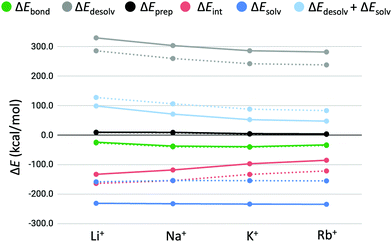Fig. 5 Partitioning of the bond energy (in kcal mol−1) between the alkali metal cations (M+) and GQ–[ ] (dashed lines) and GQ–K+–[ ] (solid lines).

In all cases, the bond energy is mainly the sum of a strong interaction energy, compensated by a large desolvation energy. The preparation energy does not vary much for the different cations and decreases upon increasing the size of the cation. This trend can be explained by looking at the cation–oxygen distance (d[O–M+]) and interplanar distance (R) (Table 2) that determine the size of the cavity hosting the second cation. The values of the geometrical parameters d[O–M+] and R come closer to the values of the empty cavity going from Li+ to Rb+. For the guanine bases to interact with the smaller cations (Li+ and Na+), the cavity should shrink. This leads to a higher preparation energy than in the case of the larger cations (K+ and Rb+), which give rise to less distortion of the empty cavity.

To investigate the contributions of geometrical effects and solvation effects, the sum of the interaction and preparation energy (ΔEint + ΔEprep) is displayed in Table 3, as well as the sum of the solvation and desolvation energy (ΔEdesolv + ΔEsolv), The former combination follows almost the exact same trend as the interaction energy alone. Table 3 shows that both summations are of counteracting and almost of equal importance to the order of cation affinity, resulting in small differences in bonding energy for the different alkali cations. The results of the double-layer GQs in Table 3 are consistent with the results found by Zaccaria et al.23 With this we would like to emphasize that the improved COSMO version results in different absolute energies, but yields the same relative energies and trends. The trends of the bond energy and the partitions are the same for both the double and triple-layer quadruplexes (Fig. 5). Remarkably, the magnitude of the bond energy decreases only little, going from the first to the second binding event. That the overall bond energy stays approximately the same for the first and second binding event of alkali metal cations of the same kind is the result of two counteracting effects: (1) the interaction energy (ΔEint) is less stabilizing for the interaction of the second metal cation (M+) with the cavity of the GQ than for the first metal cation; (2) the sum of solvation effects (ΔEdesolv + ΔEsolv) is less destabilizing for the second binding event. The latter is because the stabilizing ΔEsolv term increases more than the destabilizing ΔEdesolv term, going from the first to the second binding cation. In other words, the gain in solvation energy (ΔEsolv) for going from a GQ of charge 1+ (GQ–M+) to 2+ (GQ–K+–M+) is larger, than the increase of desolvation energy (ΔEdesolv) for going from an neutral GQ (GQ–[ ]) to a positively charged species (GQ–K+–[ ]). This can be explained by the fact that in the 2+ species the charge is more localized, resulting in more polarization of the solvent and thereupon to stronger interactions than in the case of 1+ species. It must be examined if this effect will be even stronger in highly charged systems (3+, 4+, etc.).

### 3.4 Energy decomposition analysis

The previous section showed that the desolvation energy and the size of the cation contribute equally to the bond energy of the alkali metal cations in the double and triple-layer guanine quadruplexes. The bond energy stays equal for binding a second metal cation, although a less stabilizing interaction energy was observed going from the double to the triple-layer quadruplexes. In order to get a better understanding of the reason why the interaction energy is less stabilizing for the second binding event, ΔEint is decomposed into physically meaningful terms (see eqn (7), Table 4 and Fig. 6). The interaction energy terms follow the same trend for both the guanine quadruplexes with and without sugar-phosphate backbone. The results of the systems with sugar-phosphate backbone (GQ) are graphically presented in Fig. 6.
Table 4 Partitioning of the interaction energy (in kcal mol−1) of alkali metal cations (M+) with G4–[ ]–G4, GQ–[ ], G4–K+–G4–[ ]–G4 and GQ–K+–[ ]a
System M+ ΔEint ΔVelstat ΔEPauli ΔEoi ΔEdisp
a All energies were computed with the DFT-D method at the ZORA-BLYP-D3(BJ)/TZP (G4) or at the ZORA-BLYP-D3(BJ)/TZP/DZ (GQ) level of theory.
G4M+–G4 Li+ −159.4 −108.2 11.6 −54.8 −8.0
Na+ −150.0 −105.3 11.2 −43.0 −12.9
K+ −127.2 −102.1 30.9 −40.8 −15.2
Rb+ −114.3 −96.0 36.6 −38.4 −16.5
GQ–M+ Li+ −163.6 −111.0 12.9 −58.0 −7.4
Na+ −154.2 −106.8 10.3 −44.6 −13.1
K+ −133.1 −103.6 28.4 −42.3 −15.6
Rb+ −121.5 −99.2 34.8 −40.1 −16.9
G4–K+–G4M+–G4 Li+ −119.1 −66.0 11.7 −56.1 −8.7
Na+ −105.2 −56.8 11.8 −45.3 −14.9
K+ −81.3 −51.3 30.9 −42.7 −18.2
Rb+ −68.2 −46.7 37.1 −40.3 −18.2
GQ–K+M+ Li+ −132.7 −78.6 11.8 −57.9 −8.0
Na+ −118.0 −66.7 10.1 −46.4 −15.0
K+ −96.9 −62.1 27.4 −43.7 −18.5
Rb+ −84.9 −57.6 33.4 −41.6 −19.1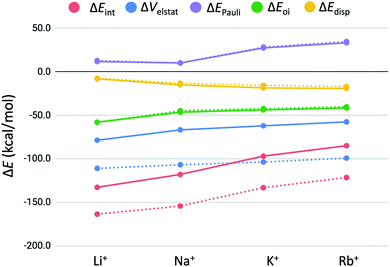Fig. 6 Energy decomposition of the interaction energy (in kcal mol−1) between the alkali metal cations (M+) and GQ–[ ] (dashed lines) and GQ–K+–[ ] (solid lines).

From Fig. 6, it can be seen that the significant drop in interaction energy going from Na+ to K+ is due to the increase in Pauli repulsion that increases by almost 20 kcal mol−1. We want to emphasize this counterintuitive observation that K+ results in less deformation of the GQ, although it is accompanied by a higher Pauli repulsion than Na+. From the energy decomposition analysis (EDA) follows that the bonding interaction of the second alkali cation to GQ–K+–[ ] is a combination of mainly electrostatic interaction, followed by orbital interaction and dispersion. These trends are also in line with the results found by Zaccaria et al.23 In fact, the trendlines of the energy terms ΔEPauli, ΔEoi and ΔEdisp do exactly overlap for the double- and triple-layered GQs. It turns out that only the decrease in electrostatic interaction (ΔVelstat) is responsible for the observed decrease in interaction energy in the second binding event.

The observed decrease in electrostatic interaction (ΔΔVelstat) for binding of the second alkali cation can be the result of the repulsion between the two present cations in the quadruplex or the diminished electron density available on middle guanine quartet to bind the second cation. The presence of the first potassium ion in GQ–K+–[ ] leads to extraction of electron density from the oxygen atoms. Thereupon, the oxygen atoms in the middle guanine quartet layer of GQ–K+M+ are likely to become less electronegative, potentially leading to less stabilizing electrostatic interaction with the second alkali cation M+.

In order to identify the effect of electron depletion of the middle guanine stack upon binding of the first alkali cation, it was examined whether there is diminished charge-transfer from the guanine bases to the second metal cation. Therefore, the VDD change in atomic charge to the second metal cation (ΔQM+) was calculated for the triple-layer GQs and compared to the values found for the two-layer GQs (eqn (8) and Table 5). As presented in Table 5, the charge-transfer interaction between the GQ and the second cation is equal to the values found for binding of the first alkali cation in two-layer GQ. This indicates that there is sufficient electron density left on the middle guanine quartet to donate the same number of electrons to the second cation. This observation is supported by the overlapping of ΔEoi (green lines in Fig. 6) for the binding of the first and second cation in the double-layer and triple-layer GQ systems, respectively.

Table 5 VDD change in atomic charge (ΔQM+, in electrons) to the alkali metal cation in the triple and double layer quadruplexesa
M+ ΔQM+ (GQ–K+M+) ΔQM+ (GQ–M+)23
a VDD charges were computed with the DFT-D method at the ZORA-BLYP-D3(BJ)/TZP/DZ level of theory.
Li+ −0.146 −0.142
Na+ −0.085 −0.079
K+ −0.059 −0.058
Rb+ −0.054 −0.054

To investigate the contribution of repulsive interactions as cause of the diminished electrostatic interaction, an EDA of the interaction energy between the two alkali cations in GQ–K+M+ was performed at the inter-atomic distance within the GQ (see Table 6). The results demonstrate that a large destabilizing interaction is present between the alkali cations in the quadruplex with interaction energies of ca. +80 kcal mol−1. Table 6 shows that this destabilizing interaction is almost exclusively due to repulsive electrostatic interactions between the two cations. The electrostatic interaction is less stabilizing by approximately +40 kcal mol−1 (ΔΔVelstat) for all the different cations in GQ–K+M+ compared to the two-layered quadruplexes (see difference in ΔVelstat in Fig. 6 for the triple-layer (solid line) and double-layer GQ (dashed line)). Therefore, it is expected that the large repulsive interaction energy between the two alkali cations contributes significantly to the observed decrease in bond energy of the second alkali metal cation but is reduced by electronic shielding of the guanine bases.

Table 6 Energy decomposition analysis (in kcal mol−1) of the interaction between the two alkali cations in GQ–K+M+a
M+ ΔEint ΔVelstat ΔEPauli ΔEoi ΔEdisp ΔΔVelstatb d[K+–M+]c
a All energies were computed with the DFT-D method at the ZORA-BLYP-D3(BJ)/TZP(/DZ) level of theory. b Difference in the electrostatic interaction component of the bond energy of GQ–K+M+vs. the two-layer GQ–M+ of Zaccaria et al.23 (see Fig. 6). c Distance (in Å) between K+ and the second alkali metal cation in GQ–K+M+.
Li+ 61.8 68.5 0.0 −0.3 −6.4 34.2 48.5
Na+ 88.1 90.2 0.0 −0.9 −1.2 42.6 36.8
K+ 86.2 88.7 0.1 −1.5 −1.2 44.2 37.5
Rb+ 83.4 86.1 0.2 −1.7 −1.1 39.5 38.5

Note that the trend in electrostatic repulsion among the different alkali metal cations is related to the distance between K+ and the second alkali metal cation in the geometry of GQ–K+M+ (d[K+–M+] in Table 6).

It can be concluded that the strong repulsive interaction between the two alkali metal cations, as found by the results in Table 6, accounts for the decrease in interaction energy of the second binding event in guanine quadruplex systems, rather than electron depletion of the middle guanine layer. This would suggest that in even larger multi-layer guanine quadruplexes, where each cation is adjacent to two other cations, the interaction energy for hosting alkali cations would decrease even further. These results question the role of alkali metal cations in the stabilization of multi-layer guanine quadruplex DNA and whether each cavity hosts an alkali metal cation or that rather an alternating pattern of empty and occupied is preferred. The decreasing importance of the alkali metal cations for the stability of multi-layer quadruplexes is in line with the results find by Kotlyar et al.53 In this work they report G4 DNA wires in the absence of alkali cations that show equal stability as the cation containing G4 structures reported in literature. The high stability is probably the result of the much greater length of these structures.

In order to see if the interaction energy for binding a third K+ ion would decrease even further, a four-layer GQ without sugar-phosphate backbone hosting three K+ ions was examined (G4–K+–G4–K+–G4–K+–G4). The usage of quadruplexes without sugar–phosphate backbone was justified by the previous results of this work. The bond energy (ΔEbond) of the third K+ ion was partitioned into different terms in the same way as was done for the triple-layer and double-layer systems (Fig. 7). It was found again that the bond energy stays approximately equal for binding of the third cation.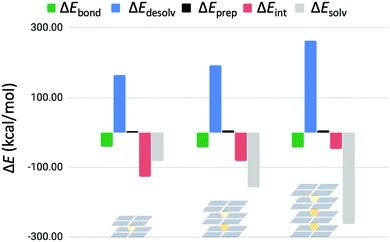Fig. 7 Partitioning of the bond energy (in kcal mol−1) for subsequent binding events of K+ in G4–K+–G4, G4–K+–G4–K+–G4 and G4–K+–G4–K+–G4–K+–G4.

In Fig. 7 the preparation energy stays the same, but the interaction energy decreases for each binding event. In addition, the contribution of solvation effects becomes more significant following this trend. The stabilizing solvation energy increases faster than the destabilizing desolvation energy with each binding event, resulting in an equalized bond energy.

The interaction energy is further decomposed into physical meaningful terms for consecutive binding events in G4K+–G4, G4–K+–G4K+–G4 and G4–K+–G4–K+–G4K+–G4 (see eqn (7) and Fig. 8). Fig. 8 shows that the energy terms ΔEPauli, ΔEoi and ΔEdisp stay the same for the subsequent binding events of K+ in double- and triple- and four-layer quadruplexes. It is only the decrease in electrostatic interaction (ΔVelstat) that is responsible for the observed decrease in interaction energy in sequel binding events. These results show that electrostatic repulsion operates not only locally, but operates also over long range distances.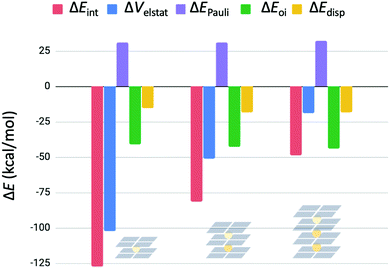Fig. 8 Energy decomposition of the interaction energy (in kcal mol−1) for subsequent binding events of K+ in G4–K+–G4, G4–K+–G4–K+–G4 and G4–K+–G4–K+–G4–K+–G4.

## 4. Conclusions

Alkali metal cations are found to stabilize the assembly of guanine quadruplexes in nature. In this work, the alkali metal cation affinity of double-, triple- and four-layer guanine quadruplexes was studied using dispersion-corrected density functional theory (DFT-D). The computational results show an increasing stabilizing interaction of the order Li+< Rb+ < Na+ < K+ for the double and triple-layer quadruplexes, which is in agreement with the experimental order of affinity.

Partitioning of the bond energy between the alkali cation and the cavity of the quadruplexes, showed that the desolvation energy and the size of the cation are of equal importance to the trend. Descending the first group of the periodic table shows a decrease of the desolvation energy of the cation, and a decrease of the attractive interaction between the cation and the quadruplex. Simultaneously, hosting of the larger cations diminishes the deformation of the empty quadruplex cavity. These effects result together in a minimum of the bond energy for K+.

The magnitude of the bond energy stays approximately the same for the subsequent binding events of a first, second and third cation in double, triple and four-layer guanine quadruplexes, respectively. Although the interaction energy decreases for each binding event, the contribution of solvation effects becomes more significant following this trend. The stabilizing solvation energy increases faster than the destabilizing desolvation energy with each binding event, resulting in an equalized bond energy.

Decomposition of the interaction energy into physically meaningful terms showed that electrostatic repulsion between the hosted metal cations is responsible for the diminished interaction energy. This work demonstrates that solvent effects become of increasing importance for the stabilization of highly charged multi-layer guanine quadruplex DNA.

## Conflicts of interest

There are no conflicts to declare.

## Acknowledgements

C. F. G. gratefully acknowledges the financial support from the Netherlands Organization for Scientific Research NWO (ECHO).

## References

1. S. Neidle and S. Balasubramanian, Quadruplex Nucleic Acids, Royal Society of Chemistry, Cambridge, 2006 Search PubMed.
2. M. L. Bochman, K. Paeschke and V. A. Zakian, Nat. Rev. Genet., 2012, 13, 770–780 CrossRef CAS.
3. R. Hänsel-Hertsch, M. Di Antonio and S. Balasubramanian, Nat. Rev. Mol. Cell Biol., 2017, 18, 279–284 CrossRef.
4. J. T. Davis, Angew. Chem., Int. Ed., 2004, 43, 668–698 CrossRef CAS.
5. E. Henderson, C. C. Hardin, S. K. Walk, I. Tinoco and E. H. Blackburn, Cell, 1987, 51, 899–908 CrossRef CAS.
6. G. Biffi, M. Di Antonio, D. Tannahill and S. Balasubramanian, Nat. Chem., 2014, 6, 75–80 CrossRef CAS.
7. S. Ray, J. N. Bandaria, M. H. Qureshi, A. Yildiz and H. Balci, Proc. Natl. Acad. Sci. U. S. A., 2014, 11, 2990–2995 CrossRef.
8. S. Neidle, Therapeutic Applications of Quadruplex Nucleic Acids, Elsevier Academic Press, 2012 Search PubMed.
9. L. Stefan and D. Monchaud, Nat. Rev. Chem., 2019, 3, 650–668 CrossRef.
10. S. Balasubramanian and S. Neidle, Curr. Opin. Chem. Biol., 2009, 13, 345–353 CrossRef CAS.
11. A. R. Duarte, E. Cadoni, A. S. Ressurreição, R. Moreira and A. Paulo, ChemMedChem, 2018, 13, 869–893 CrossRef CAS.
12. Q. Cao, Y. Li, E. Freisinger, P. Z. Qin, R. K. O. Sigel and Z. Mao, Inorg. Chem. Front., 2017, 4, 10–32 RSC.
13. C. Fonseca Guerra, H. Zijlstra, G. Paragi and F. M. Bickelhaupt, Chem. – Eur. J., 2011, 17, 12612–12622 CrossRef CAS.
14. L. P. Wolters, N. W. G. Smits and C. Fonseca Guerra, Phys. Chem. Chem. Phys., 2015, 17, 1585–1592 RSC.
15. L. Guillaumes, S. Simon and C. Fonseca Guerra, ChemistryOpen, 2015, 4, 318–327 CrossRef CAS.
16. J. Poater, M. Swart, F. M. Bickelhaupt and C. Fonseca Guerra, Org. Biomol. Chem., 2014, 12, 4691–4700 RSC.
17. C. Fonseca Guerra, F. M. Bickelhaupt and E. J. Baerends, ChemPhysChem, 2004, 5, 481–487 CrossRef.
18. C. Fonseca Guerra, Z. Szekeres and F. M. Bickelhaupt, Chem. – Eur. J., 2011, 17, 8816–8818 CrossRef CAS.
19. P. Gilli, V. Bertolasi, V. Ferretti and G. Gilli, J. Am. Chem. Soc., 2000, 122, 10405–10417 CrossRef CAS.
20. J. R. Williamson, Annu. Rev. Biophys. Biomol. Struct., 1994, 23, 703–730 CrossRef CAS.
21. J. Gu, J. Leszczynski and M. Bansal, Chem. Phys. Lett., 1999, 311, 209–214 CrossRef CAS.
22. Z. Wang and J. P. Liu, Clin. Exp. Pharmacol. Physiol., 2015, 42, 902–909 CrossRef CAS.
23. F. Zaccaria, G. Paragi and C. Fonseca Guerra, Phys. Chem. Chem. Phys., 2016, 18, 20895–20904 RSC.
24. F. Zaccaria and C. Fonseca Guerra, Chem. – Eur. J., 2018, 24, 16315–16322 CrossRef CAS.
25. R. Ida and G. Wu, J. Am. Chem. Soc., 2008, 130, 3590–3602 CrossRef CAS.
26. C. Detellier and P. Laszlo, J. Am. Chem. Soc., 1980, 102, 1135–1141 CrossRef CAS.
27. A. Wong and G. Wu, J. Am. Chem. Soc., 2003, 125, 13895–13905 CrossRef CAS.
28. J. R. Williamson, M. K. Raghuraman and T. R. Cech, Cell, 1989, 59, 871–880 CrossRef CAS.
29. J. Gu and J. Leszczynski, J. Phys. Chem. A, 2002, 106, 529–532 CrossRef CAS.
30. N. V. Hud, F. W. Smith, F. A. L. Anet and J. Feigon, Biochemistry, 1996, 35, 15383–15390 CrossRef CAS.
31. S. Grimme, J. Antony, S. Ehrlich and H. Krieg, J. Chem. Phys., 2010, 132, 154104 CrossRef.
32. S. Grimme, S. Ehrlich and L. Goerigk, J. Comput. Chem., 2011, 32, 1456–1465 CrossRef CAS.
33. S. Grimme, J. Comput. Chem., 2004, 25, 1463–1473 CrossRef CAS.
34. S. Grimme, J. Comput. Chem., 2006, 27, 1787–1799 CrossRef CAS.
35. C. Fonseca Guerra, T. van der Wijst, J. Poater, M. Swart and F. M. Bickelhaupt, Theor. Chem. Acc., 2010, 125, 245–252 Search PubMed.
36. T. van der Wijst, C. Fonseca Guerra, M. Swart, F. M. Bickelhaupt and B. Lippert, Angew. Chem., Int. Ed., 2009, 48, 3285–3287 CrossRef CAS.
37. G. N. Parkinson, M. P. H. Lee and S. Neidle, Nature, 2002, 417, 876–880 CrossRef CAS.
38. G. te Velde, F. M. Bickelhaupt, E. J. Baerends, C. Fonseca Guerra, S. J. A. van Gisbergen, J. G. Snijders and T. Ziegler, J. Comput. Chem., 2001, 22, 931–967 CrossRef CAS.
39. C. Fonseca Guerra, J. G. Snijders, G. te Velde and E. J. Baerends, Theor. Chem. Acc., 1998, 99, 391–403 Search PubMed.
40. E. J. Baerends, et al., ADF2017.103, SCM, Theoretical Chemistry, Vrije Universiteit, Amsterdam, The Netherlands http://www.scm.com.
41. E. van Lenthe, A. Ehlers and E. J. Baerends, J. Chem. Phys., 1999, 110, 8943–8953 CrossRef CAS.
42. J. Šponer, A. Mládek, N. Špačková, X. Cang, T. E. Cheatham, III and S. Grimme, J. Am. Chem. Soc., 2013, 135, 9785–9796 CrossRef.
43. R. Sedlak, T. Janowski, M. Pitoňák, J. Řezáč, P. Pulay and P. Hobza, J. Chem. Theory Comput., 2013, 9, 3364–3374 CrossRef CAS.
44. A. Klamt and G. Schüürmann, J. Chem. Soc., Perkin Trans. 2, 1993, 799–805 RSC.
45. A. Klamt, J. Phys. Chem., 1995, 99, 2224–2235 CrossRef CAS.
46. C. C. Pye, T. Ziegler, E. van Lenthe and J. N. Louwen, Can. J. Chem., 2009, 87, 790–797 CrossRef CAS.
47. F. M. Bickelhaupt and E. J. Baerends, Rev. Comput. Chem., 2002, 15, 1–86 Search PubMed.
48. C. Fonseca Guerra, J. W. Handgraaf, E. J. Baerends and F. M. Bickelhaupt, J. Comput. Chem., 2004, 25, 189–210 CrossRef.
49. O. A. Stasyuk, H. Szatylowicz, T. M. Krygowski and C. Fonseca Guerra, Phys. Chem. Chem. Phys., 2016, 18, 11624–11633 RSC.
50. C. Fonseca Guerra, F. M. Bickelhaupt, J. G. Snijders and E. J. Baerends, J. Am. Chem. Soc., 2000, 122, 4117–4128 CrossRef.
51. C. Fonseca Guerra, F. M. Bickelhaupt, J. G. Snijders and E. J. Baerends, Chem. – Eur. J., 1999, 5, 3581–3594 CrossRef.
52. T. J. Pinnavaia, C. L. Marshall, C. M. Mettler, C. L. Fisk, H. T. Miles and E. D. Becker, J. Am. Chem. Soc., 1978, 100, 3625–3627 CrossRef CAS.
53. A. B. Kotlyar, N. Borovok, T. Molotsky, H. Cohen, E. Shapir and D. Porath, Adv. Mater., 2005, 17, 1901–1905 CrossRef CAS.

### Footnote

Electronic supplementary information (ESI) available. See DOI: 10.1039/d0cp03433a
 This journal is © the Owner Societies 2020